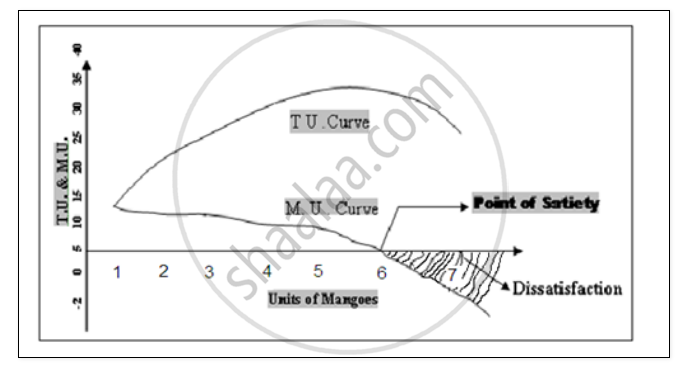# Explain the relationship between Total utility and Marginal utility. - Economics

Explain the relationship between Total utility and Marginal utility.

Discuss the relationship between total utility and marginal utility, using a hypothetical schedule.

#### Solution 1

The Relationship between Total utility and Marginal utility:-

Total utility is the sum of all utilities derived by a consumer form all units of commodity consumed by him. Whereas Marginal utility is the addition to the total utility derived by consuming an extra or additional unit of a commodity. In other words, marginal utility derived from the consumption of an additional or extra unit of a commodity.

The following illustration of a schedule and a diagram explain the relationship between total utility and Marginal utility. Let us assume that an individual consumer Mr. ‘X’ found of mangoes and start consuming unit of mangoes in quick successive unit of mangoes.

 Units of mangoes Total Utility (T.U) Marginal Utility (M.U) 1 10 10 2 18 8 3 24 6 4 28 4 5 30 2 6 30 0 7 28 -2

DiagramWith the help of the Schedule and Diagram we derives the following three conclusion:-

• Mu goes on diminishing as the consumer consumes more and more units of a commodity. And TU increases but, at a diminishing rate.
• There is an inverse relationship between MU and stock of the commodity i.e. as the stock of the commodity consumed increases, MU goes on diminishing.
• When MU is Zero, TU is the maximum and it is the point of maximum satisfaction. i.e., point of satiety.
• When Mu becomes negative, total utility starts diminishing. This is the area of dissatisfaction.

#### Solution 2

Total Utility refers to the aggregate utility or summation of utility derived from the consumption of all the units of a commodity. Algebraically, TU = TU1 + TU2 + TU3 + ...............+ TUn

The given is the schedule which shows the relationship between total utility and marginal utility.

 Number of Units Consumed of Commodity X Total Utility (TU)(utils) Marginal Utility (MU)MUn = TUn – TUn – 1(utils) 1 50 50 – 0 = 50 2 100 100 – 50 = 50 3 130 130 – 100 = 30 4 150 150 – 130 = 20 5 160 160 – 150 = 10 6 160 160 – 160 = 0 7 150 150 – 160 = – 10
1. As more and more units of the commodity are consumed, the Marginal Utility derived from the consumption of each additional unit of the commodity tends to fall. With the consumption of the successive units, the Marginal Utility becomes zero and consequently becomes negative. (As per the schedule, MU is zero at the consumption of 6th unit and becomes negative at 7th unit consumed).
2. As long as MU derived from the consumption of additional units of the commodity is positive, TU continues to rise. (MU is positive till the consumption of the 5th unit of the commodity.)
3. When TU becomes maximum (also known as Saturation Point), MU becomes zero. (TU is maximum at 160 utils and MU is zero at the 6th unit.)
4. When TU starts falling, MU becomes negative. (For the consumption of 7th unit, MU becomes negative and accordingly, the TU falls from 160 utils to 150 utils).
Concept: Total Utility and Marginal Utility
Is there an error in this question or solution?